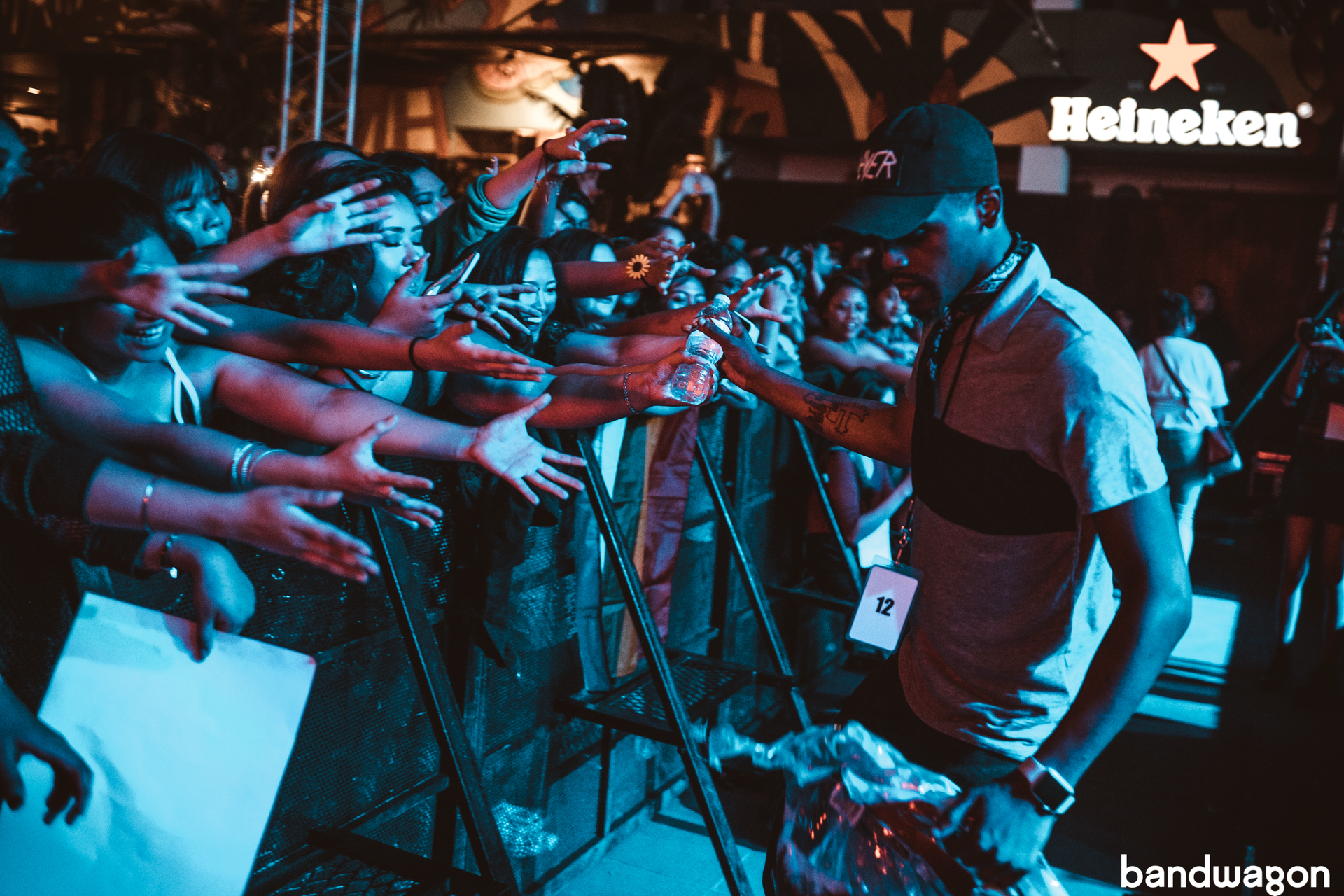# Kehlani in Manila: "I will remember this. I will remember y'all."Grammy nominated R&B singer Kehlani's first visit to Manila was marked with a warm reception served by her sea of fans and the 90-degree Manila heat. Her dazzlingly pitch-perfect voice and sultry moves was on full force for the entire hour and a half duration of the concert. Meanwhile, The Island -- formerly known as Palace Pool Club, served as the perfect venue for Kehlani to turn the burn a notch higher on the already sweltering summer heat.

On tour for her album, SweetSexySavage, it was no surprise that her setlist mostly comprised of her more recent bops but she treated older fans by performing two songs from her 2014 mixtape Wish You Were Here, 'The Way' and 'Wish You Were Here'.

The 23 year old singer has always been vocal about inclusiveness and intolerance for violence which she lived out wholly as she, without hesitation, asked a disruptive group in the audience to be escorted out of the venue.

She brushed it off quickly and gave her attention back to her adoring fans which she spoke to conversationally, building a relationship which she hopes to return to for a future concert. “One day, when I come back here and I [will] be playin’ in an arena, I will remember this. I will remember y’all,” she promised.

In return, the crowd sang along to her every word and fan favorite, 'Honey', was specially dedicated to the LGBTQ+ community. “Whatever you do, no matter where you go, whatever it is you believe in, you have to rep that shit now,” as she stirs the crowd with the spirit of inclusiveness and individuality. "Stick up for people who cannot stick up for themselves. You be loud for those who don’t have a voice."

Check out the highlights from her show:### The singer's production team showed their concern to the fans by giving away water and making sure everyone stayed hydrated.## window.NREUM||(NREUM={});NREUM.info={"beacon":"bam.nr-data.net","errorBeacon":"bam.nr-data.net","licenseKey":"c44b183b27","applicationID":"7377923","transactionName":"dlgNRhNYW15SSxpSE0FeAF4ERBhBX1ZC","queueTime":14,"applicationTime":279,"agent":""}(window.NREUM||(NREUM={})).loader_config={licenseKey:"c44b183b27",applicationID:"7377923"};window.NREUM||(NREUM={}),__nr_require=function(e,t,n){function r(n){if(!t[n]){var i=t[n]={exports:{}};e[n].call(i.exports,function(t){var i=e[n][t];return r(i||t)},i,i.exports)}return t[n].exports}if("function"==typeof __nr_require)return __nr_require;for(var i=0;i<n.length;i++)r(n[i]);return r}({1:[function(e,t,n){function r(){}function i(e,t,n){return function(){return o(e,[u.now()].concat(c(arguments)),t?null:this,n),t?void 0:this}}var o=e("handle"),a=e(7),c=e(8),f=e("ee").get("tracer"),u=e("loader"),s=NREUM;"undefined"==typeof window.newrelic&&(newrelic=s);var d=["setPageViewName","setCustomAttribute","setErrorHandler","finished","addToTrace","inlineHit","addRelease"],p="api-",l=p+"ixn-";a(d,function(e,t){s[t]=i(p+t,!0,"api")}),s.addPageAction=i(p+"addPageAction",!0),s.setCurrentRouteName=i(p+"routeName",!0),t.exports=newrelic,s.interaction=function(){return(new r).get()};var m=r.prototype={createTracer:function(e,t){var n={},r=this,i="function"==typeof t;return o(l+"tracer",[u.now(),e,n],r),function(){if(f.emit((i?"":"no-")+"fn-start",[u.now(),r,i],n),i)try{return t.apply(this,arguments)}catch(e){throw f.emit("fn-err",[arguments,this,e],n),e}finally{f.emit("fn-end",[u.now()],n)}}}};a("actionText,setName,setAttribute,save,ignore,onEnd,getContext,end,get".split(","),function(e,t){m[t]=i(l+t)}),newrelic.noticeError=function(e,t){"string"==typeof e&&(e=new Error(e)),o("err",[e,u.now(),!1,t])}},{}],2:[function(e,t,n){function r(){return c.exists&&performance.now?Math.round(performance.now()):(o=Math.max((new Date).getTime(),o))-a}function i(){return o}var o=(new Date).getTime(),a=o,c=e(9);t.exports=r,t.exports.offset=a,t.exports.getLastTimestamp=i},{}],3:[function(e,t,n){function r(e){return!(!e||!e.protocol||"file:"===e.protocol)}t.exports=r},{}],4:[function(e,t,n){function r(e,t){var n=e.getEntries();n.forEach(function(e){"first-paint"===e.name?d("timing",["fp",Math.floor(e.startTime)]):"first-contentful-paint"===e.name&&d("timing",["fcp",Math.floor(e.startTime)])})}function i(e,t){var n=e.getEntries();n.length>0&&d("lcp",[n[n.length-1]])}function o(e){e.getEntries().forEach(function(e){e.hadRecentInput||d("cls",[e])})}function a(e){if(e instanceof m&&!g){var t=Math.round(e.timeStamp),n={type:e.type};t<=p.now()?n.fid=p.now()-t:t>p.offset&&t<=Date.now()?(t-=p.offset,n.fid=p.now()-t):t=p.now(),g=!0,d("timing",["fi",t,n])}}function c(e){d("pageHide",[p.now(),e])}if(!("init"in NREUM&&"page_view_timing"in NREUM.init&&"enabled"in NREUM.init.page_view_timing&&NREUM.init.page_view_timing.enabled===!1)){var f,u,s,d=e("handle"),p=e("loader"),l=e(6),m=NREUM.o.EV;if("PerformanceObserver"in window&&"function"==typeof window.PerformanceObserver){f=new PerformanceObserver(r);try{f.observe({entryTypes:["paint"]})}catch(v){}u=new PerformanceObserver(i);try{u.observe({entryTypes:["largest-contentful-paint"]})}catch(v){}s=new PerformanceObserver(o);try{s.observe({type:"layout-shift",buffered:!0})}catch(v){}}if("addEventListener"in document){var g=!1,w=["click","keydown","mousedown","pointerdown","touchstart"];w.forEach(function(e){document.addEventListener(e,a,!1)})}l(c)}},{}],5:[function(e,t,n){function r(e,t){if(!i)return!1;if(e!==i)return!1;if(!t)return!0;if(!o)return!1;for(var n=o.split("."),r=t.split("."),a=0;a<r.length;a++)if(r[a]!==n[a])return!1;return!0}var i=null,o=null,a=/Version\/(\S+)\s+Safari/;if(navigator.userAgent){var c=navigator.userAgent,f=c.match(a);f&&c.indexOf("Chrome")===-1&&c.indexOf("Chromium")===-1&&(i="Safari",o=f)}t.exports={agent:i,version:o,match:r}},{}],6:[function(e,t,n){function r(e){function t(){e(a&&document[a]?document[a]:document[i]?"hidden":"visible")}"addEventListener"in document&&o&&document.addEventListener(o,t,!1)}t.exports=r;var i,o,a;"undefined"!=typeof document.hidden?(i="hidden",o="visibilitychange",a="visibilityState"):"undefined"!=typeof document.msHidden?(i="msHidden",o="msvisibilitychange"):"undefined"!=typeof document.webkitHidden&&(i="webkitHidden",o="webkitvisibilitychange",a="webkitVisibilityState")},{}],7:[function(e,t,n){function r(e,t){var n=[],r="",o=0;for(r in e)i.call(e,r)&&(n[o]=t(r,e[r]),o+=1);return n}var i=Object.prototype.hasOwnProperty;t.exports=r},{}],8:[function(e,t,n){function r(e,t,n){t||(t=0),"undefined"==typeof n&&(n=e?e.length:0);for(var r=-1,i=n-t||0,o=Array(i<0?0:i);++r<i;)o[r]=e[t+r];return o}t.exports=r},{}],9:[function(e,t,n){t.exports={exists:"undefined"!=typeof window.performance&&window.performance.timing&&"undefined"!=typeof window.performance.timing.navigationStart}},{}],ee:[function(e,t,n){function r(){}function i(e){function t(e){return e&&e instanceof r?e:e?u(e,f,a):a()}function n(n,r,i,o,a){if(a!==!1&&(a=!0),!l.aborted||o){e&&a&&e(n,r,i);for(var c=t(i),f=v(n),u=f.length,s=0;s<u;s++)f[s].apply(c,r);var p=d[h[n]];return p&&p.push([b,n,r,c]),c}}function o(e,t){y[e]=v(e).concat(t)}function m(e,t){var n=y[e];if(n)for(var r=0;r<n.length;r++)n[r]===t&&n.splice(r,1)}function v(e){return y[e]||[]}function g(e){return p[e]=p[e]||i(n)}function w(e,t){s(e,function(e,n){t=t||"feature",h[n]=t,t in d||(d[t]=[])})}var y={},h={},b={on:o,addEventListener:o,removeEventListener:m,emit:n,get:g,listeners:v,context:t,buffer:w,abort:c,aborted:!1};return b}function o(e){return u(e,f,a)}function a(){return new r}function c(){(d.api||d.feature)&&(l.aborted=!0,d=l.backlog={})}var f="nr@context",u=e("gos"),s=e(7),d={},p={},l=t.exports=i();t.exports.getOrSetContext=o,l.backlog=d},{}],gos:[function(e,t,n){function r(e,t,n){if(i.call(e,t))return e[t];var r=n();if(Object.defineProperty&&Object.keys)try{return Object.defineProperty(e,t,{value:r,writable:!0,enumerable:!1}),r}catch(o){}return e[t]=r,r}var i=Object.prototype.hasOwnProperty;t.exports=r},{}],handle:[function(e,t,n){function r(e,t,n,r){i.buffer([e],r),i.emit(e,t,n)}var i=e("ee").get("handle");t.exports=r,r.ee=i},{}],id:[function(e,t,n){function r(e){var t=typeof e;return!e||"object"!==t&&"function"!==t?-1:e===window?0:a(e,o,function(){return i++})}var i=1,o="nr@id",a=e("gos");t.exports=r},{}],loader:[function(e,t,n){function r(){if(!E++){var e=x.info=NREUM.info,t=l.getElementsByTagName("script");if(setTimeout(u.abort,3e4),!(e&&e.licenseKey&&e.applicationID&&t))return u.abort();f(h,function(t,n){e[t]||(e[t]=n)});var n=a();c("mark",["onload",n+x.offset],null,"api"),c("timing",["load",n]);var r=l.createElement("script");r.src="https://"+e.agent,t.parentNode.insertBefore(r,t)}}function i(){"complete"===l.readyState&&o()}function o(){c("mark",["domContent",a()+x.offset],null,"api")}var a=e(2),c=e("handle"),f=e(7),u=e("ee"),s=e(5),d=e(3),p=window,l=p.document,m="addEventListener",v="attachEvent",g=p.XMLHttpRequest,w=g&&g.prototype;if(d(p.location)){NREUM.o={ST:setTimeout,SI:p.setImmediate,CT:clearTimeout,XHR:g,REQ:p.Request,EV:p.Event,PR:p.Promise,MO:p.MutationObserver};var y=""+location,h={beacon:"bam.nr-data.net",errorBeacon:"bam.nr-data.net",agent:"js-agent.newrelic.com/nr-1208.min.js"},b=g&&w&&w[m]&&!/CriOS/.test(navigator.userAgent),x=t.exports={offset:a.getLastTimestamp(),now:a,origin:y,features:{},xhrWrappable:b,userAgent:s};e(1),e(4),l[m]?(l[m]("DOMContentLoaded",o,!1),p[m]("load",r,!1)):(l[v]("onreadystatechange",i),p[v]("onload",r)),c("mark",["firstbyte",a.getLastTimestamp()],null,"api");var E=0}},{}],"wrap-function":[function(e,t,n){function r(e,t){function n(t,n,r,f,u){function nrWrapper(){var o,a,s,p;try{a=this,o=d(arguments),s="function"==typeof r?r(o,a):r||{}}catch(l){i([l,"",[o,a,f],s],e)}c(n+"start",[o,a,f],s,u);try{return p=t.apply(a,o)}catch(m){throw c(n+"err",[o,a,m],s,u),m}finally{c(n+"end",[o,a,p],s,u)}}return a(t)?t:(n||(n=""),nrWrapper[p]=t,o(t,nrWrapper,e),nrWrapper)}function r(e,t,r,i,o){r||(r="");var c,f,u,s="-"===r.charAt(0);for(u=0;u<t.length;u++)f=t[u],c=e[f],a(c)||(e[f]=n(c,s?f+r:r,i,f,o))}function c(n,r,o,a){if(!m||t){var c=m;m=!0;try{e.emit(n,r,o,t,a)}catch(f){i([f,n,r,o],e)}m=c}}return e||(e=s),n.inPlace=r,n.flag=p,n}function i(e,t){t||(t=s);try{t.emit("internal-error",e)}catch(n){}}function o(e,t,n){if(Object.defineProperty&&Object.keys)try{var r=Object.keys(e);return r.forEach(function(n){Object.defineProperty(t,n,{get:function(){return e[n]},set:function(t){return e[n]=t,t}})}),t}catch(o){i([o],n)}for(var a in e)l.call(e,a)&&(t[a]=e[a]);return t}function a(e){return!(e&&e instanceof Function&&e.apply&&!e[p])}function c(e,t){var n=t(e);return n[p]=e,o(e,n,s),n}function f(e,t,n){var r=e[t];e[t]=c(r,n)}function u(){for(var e=arguments.length,t=new Array(e),n=0;n<e;++n)t[n]=arguments[n];return t}var s=e("ee"),d=e(8),p="nr@original",l=Object.prototype.hasOwnProperty,m=!1;t.exports=r,t.exports.wrapFunction=c,t.exports.wrapInPlace=f,t.exports.argsToArray=u},{}]},{},["loader"]);### The crowd was hyped up by Kehlani’s touring dj, DJ Noodles.### Fans who were eagerly waiting as early as 8 p.m. brought gifts such as fan art and symbols of support such as the rainbow flag, which Kehlani gratefully accepted in between performances.### "Stick up for people who cannot stick up for themselves. You be loud for those who don’t have a voice."### The Manila crowd showing nothing but love for Kehlani.Kehlani in Manila was presented by MyMusicTaste.